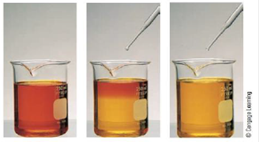# Chromium(VI) forms two different oxyanions, the orange dichromate ion, Cr 2 O 7 2− , and the yellow chromate ion, CrO 4 2− . (See the following photos.) The equilibrium reaction between the two ions is Cr 2 O 7 2 − ( a q ) + H 2 O ( l ) ⇌ 2 CrO 4 2 − ( a q ) + 2 H + ( a q ) Explain why orange dichromate solutions turn yellow when sodium hydroxide is added.### Chemistry: An Atoms First Approach

2nd Edition
Steven S. Zumdahl + 1 other
Publisher: Cengage Learning
ISBN: 9781305079243

#### Solutions

Chapter
Section### Chemistry: An Atoms First Approach

2nd Edition
Steven S. Zumdahl + 1 other
Publisher: Cengage Learning
ISBN: 9781305079243
Chapter 12, Problem 83AE
Textbook Problem
49 views

## Chromium(VI) forms two different oxyanions, the orange dichromate ion, Cr2O72− , and the yellow chromate ion, CrO42− .(See the following photos.) The equilibrium reaction between the two ions is Cr 2 O 7 2 − ( a q ) + H 2 O ( l ) ⇌ 2 CrO 4 2 − ( a q ) + 2 H + ( a q ) Explain why orange dichromate solutions turn yellow when sodium hydroxide is added.Interpretation Introduction

Interpretation: The color and reaction between chromium (VI) oxyanions is given. The reason of change in color of dichromate solution by the addition of sodium hydroxide is to be given.

Concept introduction: The anion that contains oxygen atoms is known as oxyanion.

According to Le Chatelier’s principle, “When equilibrium is subjected to change in variable, the shift in the equilibrium is produced in the direction that nullifies the effect of change”.

To explain: The reason that orange dichromate solution turns yellow when sodium hydroxide is added.

### Explanation of Solution

Explanation

The given reaction is,

Cr2O72(aq)+H2O(l)2CrO42(aq)+2H+(aq)

Chromium (VI) forms two different oxyanions, the orange dichromate ion (Cr2

### Still sussing out bartleby?

Check out a sample textbook solution.

See a sample solution

#### The Solution to Your Study Problems

Bartleby provides explanations to thousands of textbook problems written by our experts, many with advanced degrees!

Get Started

Find more solutions based on key concepts
Most water-soluble vitamins are not stored in tissues to any great extent. T F

Nutrition: Concepts and Controversies - Standalone book (MindTap Course List)

What is a consensus sequence in transcription?

Introduction to General, Organic and Biochemistry

A line of positive charge is formed into a semicircle of radius R = 60.0 cm as shown in Figure P23.41. The char...

Physics for Scientists and Engineers, Technology Update (No access codes included)